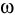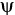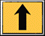# Computational Science for the 21st century

## Conference in honor of Professor Roland Glowinski on the occasion of his 60th birthday

May 5-7, 1997
Tours (France)
Organization:
INRIA, University of Houston and GAMNI/SMAI

CONTENTS OF THE CONFERENCE BOOK:

COMPUTATIONAL SCIENCE FOR THE 21ST CENTURY

Edited by
M-O. Bristeau, INRIA Rocquencourt, Le Chesnay, France
G. Etgen, University of Houston, USA
W. Fitzgibbon, University of Houston, USA
J.L. Lions, Collège de France, Paris, France
J. Périaux, Dassault Aviation, Saint Cloud, France
M.F. Wheeler, University of Texas at Austin, USA

Computational science can loosely be defined as the endeavour to develop and analyse models for the simulation and control of complex processes. This is achieved by making optimal use of computer resources and by drawing upon a variety of disciplines, techniques and theories. With the emergence of advanced computational and information technologies, computational science now has a significant impact on engineering, physical, biological, management and social sciences.

The contributions in this state-of-the-art volume range from theoretical and numerical topics to practical implementations. The subject matter includes modelling, mathematical and numerical analysis, differential equations, linear algebra, optimization, domain decomposition, computational fluid dynamics, computational mechanics, elasticity, structures, computational physics and chemistry, electromagnetics, control theory and other applications.

This volume is dedicated to Roland Glowinski on the occasion of his 60th birthday. It is aimed at the next generation of scientists, applied mathematicians, computer scientists, practitioners and engineers who will define computational science within the context of the challenging scientific, industrial, economic and societal problems of the 21st century.

Published by JOHN WILEY & SONS
ISBN 0.471.97.298.3

## Contents

Preface

List of authors

CHAPTER 1 MATHEMATICAL ANALYSIS

Remarks about metastability
R. Jordan, D. Kinderlehrer, F. Otto

Global solutions to the system of the motion of elastic strings
L. Ta-tsien, L. Da-qian

Regularity results for the solution of the Cauchy problem to Navier-Stokes equations
J. Necas

Analyticity, and the lack thereof, of semigroups arising from thermo-elastic plates
R. Triggiani

CHAPTER 2 NUMERICAL LINEAR ALGEBRA

Roundoff induces a chaotic behaviour for eigensolvers applied to highly nonnormal matrices
T. Braconnier, F. Chaitin-Chatelin

About Newton-Krylov methods
J. Erhel

New approaches to large scale eigenanalysis
D. C. Sorensen

An approach to continuation using Krylov subspace methods
H. F. Walker

CHAPTER 3 MULTIGRIDS, DOMAIN DECOMPOSITION & FICTITIOUS DOMAIN METHODS

Adaptive algorithms and a posteriori error estimates on partitioned meshes
R. E. Bank, J. Périaux

Parallel domain decomposition for reaction-diffusion problems
M. Bercovier, N. Volfovsky, H. Parnas

Parallel solutions of optimal-control problems by time-domain decomposition
M. Berggren,M. Heinkenschloss

An analytic basis for multigrid methods for the stabilized finite element methods for the Stokes problem
Z. Cai, J. Douglas Jr

Numerical quadratures and mortar methods
L. Cazabeau, C. Lacour, Y. Maday

Local inexact Newton multilevel FEM for nonlinear elliptic problems
P. Deuflhard, M. Weiser

An analytical study of the optimal speed of convergence of iterations in DDM under certain shape assumptions of domains
H. Fujita, N. Saito

A fictitious domain method for Navier-Stokes equations
V. Girault, R. Glowinski, H. López, J.-P. Vila

Parallel solution of the wave equation using higher order finite elements
M. Kern

Iterative analysis of finite element problems with Lagrange multipliers
Y. Kuznetsov

On embedding techniques for 2nd-order elliptic problems
A. Rieder

Sharp estimates on intergrid transfer operators for P1-nonconforming element and Morley element
Z. Shi, Z. Xie

Non-overlapping domain decomposition methods
R. Verfürth

CHAPTER 4 NUMERICAL SCHEMES & CFD

A non-oscillatory modified method of characteristics algorithm
R. Bermejo

On the self-stabilization of Galerkin-finite element methods for flow problems
T. Chacon-Rebollo, A. D. Delgado

Generalized cell-centered finite volume methods: application to two-phase flow in porous media
G. Chavent, J. Jaffré, J.E. Roberts

On an operator related to the convergence of Uzawa's algorithm for the Stokes equation
M. Crouzeix

Computational sciences in environmental applications
R. E. Ewing

Remarks on a parallel algorithm for the Navier-Stokes equations
E. Fernández-Cara

Distributed Lagrange multiplier methods for particulate flows
R. Glowinski, T. Hesla, D. D. Joseph, T.-W. Pan, J. Périaux

Glowinski-Pironneau method for 3D-equations
J.-L. Guermond, L. Quartapelle

A preconditioned GMRES algorithm for compressible and incompressible flows
S. Idelsohn, N. Nigro, M. Storti

Numerical solution of the incompressible fluid flows using lattice Boltzmann method
N. Satofuka, T. Nishioka

A fluid particle motion simulation method
A.-K. Tornberg, R. W. Metcalfe, R. Scott, B. Bagheri

CHAPTER 5 APPLICATIONS & CFD

Numerical experiments on non-equilibrium effects in rarefied high velocity flows
J.-F. Bourgat

Numerical simulation of turbulent flows on unstructured meshes by using finite element methods
J.-P. Chabard, F. Archambeau, O. Bonnin, D. Laurence, G. Pot

The simulation of 3D unsteady inviscid compressible flows with moving boundaries
K. Morgan, L. B. Bayne, O. Hassan, E. J. Probert, N. P. Weatherill

Some problems and paths to next century unsteady multiscale CFD
P. Perrier

Modeling and simulation of blood flow problems
A. Quarteroni, A. Veneziani

Turbulence modeling
M. Ravachol

A few issues of future simulation tools in computational fluid dynamics for aeronautical applications
B. Stoufflet

CHAPTER 6 COMPUTATIONAL PHYSICS & CHEMISTRY

Thermal diffusion effects in multicomponent flows
A. Ern, V. Giovangigli

Analysis and numerical simulation for models of binary alloy solidification
F. Gaillard, J. Rappaz

Some mathematical and numerical aspects of reduction in chemical kinetics
B. Larrouturou, B. Sportisse

Computational challenges in theoretical chemistry
B. M. Pettitt, P.E. Smith

Variational methods for biomedical computing
W. Lawton, N. Lek, T. Poston, R. Raghavan, S. R. Ranjan, R. Viswanathan, Y. P. Wang, Y. Yu

Coupling 1D and 2D mixed simulation of heterogeneous semiconductor devices
A. Marrocco

CHAPTER 7 COMPUTATIONAL ELECTROMAGNETICS

Thermoelectrical simulation of electrodes for reduction furnaces
A. Bermúdez, J. Bullón, F. Pena

Optimal shape design for radar cross section minimisation: the case of cylindrical bodies
J. Blum, M. Mandallena

Exact controllability methods for calculation of 3D time-periodic Maxwell solutions
M.-O. Bristeau

An asymptotic approach of the scattering of electromagnetic waves by thin coatings with ferromagnetic materials
P. Joly, C. Poirier

Application of exact controllability and genetic algorithms to the problem of scattering waves
J. Periaux, B. Mantel, H. Q. Chen

CHAPTER 8 COMPUTATIONAL ELASTICITY & STRUCTURES

A criterion for stability of structures in the neighbourhood of a limit-point
E. Absi, A. Rigolot

Homogenization of a transmission problem in 2D elasticity
L. Baffico, C. Conca

On the role of the interface in the constructive or approximate solution of junction problems
R. L. V. González, E. Rofman

Convergence rates for semidiscrete FEM approximations of dynamic nonlinear shallow shells
I. Lasiecka, R. Marchand

Domain decomposition techniques for nonlinear elasticity problems
P. Le Tallec, M. Vidrascu

CHAPTER 9 OPTIMIZATION

Investigation of a class of inverse problems on optimal boundaries
V. Agoshkov

Augmented Lagrangian and total variation methods for recovering discontinuous coefficients from elliptic equations
T. F. Chan, X.-C. Tai

Aspects of optimal control on aerodynamics
A. Dervieux, J.-A. Désidéri, N. Marco

Aerodynamic design
A. Jameson

Some relations between two strategies for solving optimal control problems with bilinear constraints
T. Kärkkäinen, P. Neittaanmäki

Fuzzy optimization method
H. Kawarada, H. Suito

CHAPTER 10 CONTROL

Control theoretic methods for the computation of special solutions of differential equations
G. Auchmuty

Smart structures and super stability
A. V. Balakrishnan

Penalization methods applied to nonlinear elliptic unilateral problems
A. Bensoussan, L. Boccardo

Approximate controllability and obstruction phenomena for quasilinear diffusion equations
J. I. Díaz, A. M. Ramos

A rapid prototyping workstation for design of distributed parameter control systems
C. LaVigna, M. Mattice, H. G. Kwatny, G. L. Blankenship

On the approximate controllability with global state constraints
J. L. Lions

Sensitivity of functionals
G. Marchuk

Approximate controllability of the semilinear heat equation: boundary control
E. Zuazua

CHAPTER 11 COMPUTERS & SYSTEMS

On numerical schemes for computing the price of look-back options
G. Barles, C. Daher, M. Romano, C. Tezier

An industrial vision of scientific computation for the 21st century
P. Bohn

Crisis or mutation in high-performance computing
P. Caseau

Filtering techniques in parallel computing
A. Ecer, H. U. Akay, N. Gopalaswamy

The description of the geographic spread in infectious disease
W. E. Fitzgibbon, M. Langlais

Vector finite elements and C++
F. Hecht, O. Pironneau

Numerical studies of the Gauss lattice problem
H. B. Keller

A robust resolution for the Zakai equation and infinitesimal generator of the asymptotic filtering process
P. Malliavin

Coifman wavelet systems: approximation, smoothness and computational algorithms
J. Tian, R. O. Wells Jr, J. E. Odergard, C. S. BurrusINRIA Ucis